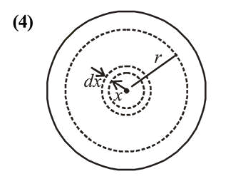# The mass density of a planet of radius $R$ varies with theQuestion:

The mass density of a planet of radius $R$ varies with the distance $r$ from its centre as $\rho(r)=\rho_{0}\left(1-\frac{r^{2}}{R^{2}}\right)$. Then the

gravitational field is maximum at:

1. (1) $r=\sqrt{\frac{3}{4}} R$

2. (2) $r=R$

3. (3) $r=\frac{1}{\sqrt{3}} R$

4. (4) $r=\sqrt{\frac{5}{9}} R$

Correct Option: , 4

Solution:Mass of small element of planet of radius $x$ and thickness $d x$.

$d m=\rho \times 4 \pi x^{2} d x=\rho_{0}\left(1-\frac{x^{2}}{R^{2}}\right) \times 4 \pi x^{2} d x$

Mass of the planet

$M=4 \pi \rho_{0} \int_{0}^{r}\left(x^{2}-\frac{x^{4}}{R^{2}}\right) d x$

$\Rightarrow M=4 \pi \rho_{0}\left|\frac{r^{3}}{3}-\frac{r^{5}}{5 R^{2}}\right|$

Gravitational field,

$E=\frac{G M}{r^{2}}=\frac{G}{r^{2}} \times 4 \pi \rho_{0}\left(\frac{r^{3}}{3}-\frac{r^{5}}{5 R^{2}}\right)$

$\Rightarrow E=4 \pi G \rho_{0}\left(\frac{r}{3}-\frac{r^{3}}{5 R^{2}}\right)$

$E$ is maximum when $\frac{d E}{d r}=0$

$\Rightarrow \frac{d E}{d r}=4 \pi G \rho_{0}\left(\frac{1}{3}-\frac{3 r^{2}}{5 R^{2}}\right)=0$

$\Rightarrow r=\frac{\sqrt{5}}{3} R$